Next: Non-bonded interactions Up: Force Field Parameters Previous: Force Field Parameters

Subsections

## Potential energy functions

Evaluating the force is the most computationally demanding part of molecular dynamics. The force is the negative gradient of a scalar potential energy function,(1)

and, for systems of biomolecules, this potential function involves the summing,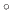(2)

over a large number of bonded and nonbonded terms. The bonded potential terms involve 2-, 3-, and 4-body interactions of covalently bonded atoms, withterms in the summation. The nonbonded potential terms involve interactions between all pairs of atoms (usually excluding pairs of atoms already involved in a bonded term), with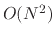terms in the summation, although fast evaluation techniques are used to compute good approximations to their contribution to the potential withor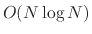computational cost.

### Bonded potential energy terms

The bonded potential terms involve 2-, 3-, and 4-body interactions of covalently bonded atoms.

The 2-body spring bond potential describes the harmonic vibrational motion between an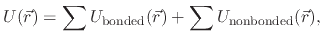-pair of covalently bonded atoms,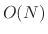(3)

wheregives the distance between the atoms,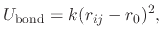is the equilibrium distance, andis the spring constant.

The 3-body angular bond potential describes the angular vibrational motion occurring between an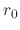-triple of covalently bonded atoms,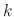(4)

where, in the first term,is the angle in radians between vectors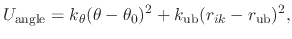and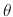,is the equilibrium angle, and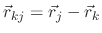is the angle constant. The second term is the Urey-Bradley term used to describe a (noncovalent) spring between the outer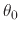andatoms, active when constant, where, like the spring bond,gives the distance between the pair of atoms andis the equilibrium distance.

The 4-body torsion angle (also known as dihedral angle) potential describes the angular spring between the planes formed by the first three and last three atoms of a consecutively bonded-quadruple of atoms,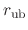(5)

whereis the angle in radians between the-plane and the-plane. The integer constant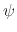is nonnegative and indicates the periodicity. For,is the phase shift angle andis the multiplicative constant. For,acts as an equilibrium angle and the units ofchange to potential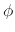rad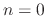. A given-quadruple of atoms might contribute multiple terms to the potential, each with its own parameterization. The use of multiple terms for a torsion angle allows for complex angular variation of the potential, effectively a truncated Fourier series.

### Nonbonded potential energy terms

The nonbonded potential terms involve interactions between all-pairs of atoms, usually excluding pairs of atoms already involved in a bonded term. Even using a fast evaluation methods the cost of computing the nonbonded potentials dominates the work required for each time step of an MD simulation.

The Lennard-Jones potential accounts for the weak dipole attraction between distant atoms and the hard core repulsion as atoms become close,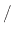(6)

wheregives the distance between the pair of atoms. The parameter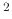is the minimum of the potential term (, which means that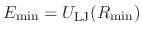is the well-depth). The Lennard-Jones potential approaches 0 rapidly asincreases, so it is usually truncated (smoothly shifted) to 0 past a cutoff radius, requiringcomputational cost.

The electrostatic potential is repulsive for atomic charges with the same sign and attractive for atomic charges with opposite signs,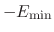(7)

wheregives the distance between the pair of atoms, and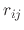and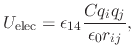are the charges on the respective atoms. Coulomb's constantand the dielectric constant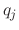are fixed for all electrostatic interactions. The parameter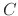is a unitless scaling factor whose value is 1, except for a modified 1-4 interaction, where the pair of atoms is separated by a sequence of three covalent bonds (so that the atoms might also be involved in a torsion angle interaction), in which case, for a fixed constant. Although the electrostatic potential may be computed with a cutoff like the Lennard-Jones potential, the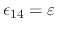potential approaches 0 much more slowly than the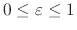potential, so neglecting the long range electrostatic terms can degrade qualitative results, especially for highly charged systems. There are other fast evaluation methods that approximate the contribution to the long range electrostatic terms that requireorcomputational cost, depending on the method.Next: Non-bonded interactions Up: Force Field Parameters Previous: Force Field Parameters
http://www.ks.uiuc.edu/Research/namd/